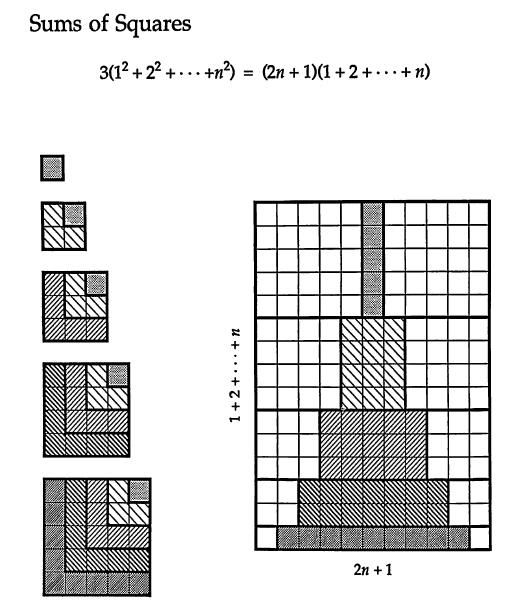# Thread: Summation proof

1. ## Summation proof

Hey all, any help proving the following summation would be greatly appreciated!

Σ from j=1 to k of j^2 = k(k+1)(2k+1) / 6

Thank you!

2.Originally Posted by jstarks44444Hey all, any help proving the following summation would be greatly appreciated!

Σ from j=1 to k of : k(k+1)(2k+1) / 6
Have you written what you meant?

3.Originally Posted by jstarks44444Hey all, any help proving the following summation would be greatly appreciated!

Σ from j=1 to k of j^2 = k(k+1)(2k+1) / 6

Thank you!
See...

http://www.mathhelpforum.com/math-he...la-172620.html

... taking into account that is $\displaystyle \displaystyle S_{1,n}= \frac{n\ (n+1)}{2}$ ...

Kind regards

$\displaystyle \chi$ $\displaystyle \sigma$

4.#### Search Tags

proof, summation